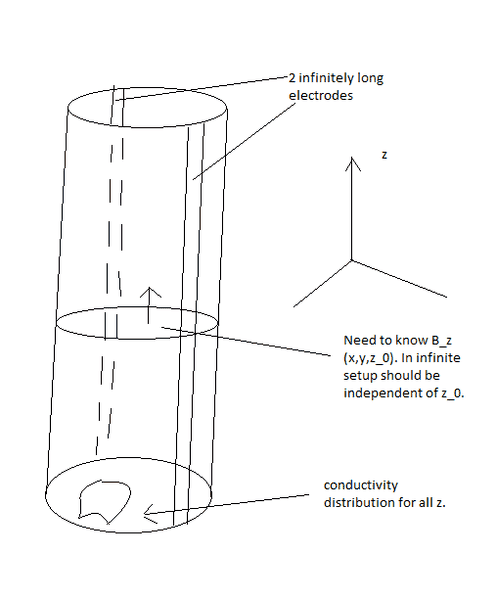# Infinite cylindrical conductor - calculating B_z

Rholle
Hello people,

I am doing some work where I need to look at a simplified situation regarding a conductor for which the conductivity distribution does not change along the z-direction of an infinite cylinder. The distribution itself is not symmetric in any way. Presume 2 infinitely long electrodes along the z-direction with I_1 = - I_2 at every point along those electrodes. Thus the current density J = J(x,y) is independent of z.

I am looking to simplify the calculation of the z-component of the resulting magnetic field inside the conductor in a slice, z_0, and hoping that I do not need the full volume integration of the Biot-Savart law.

Anyone got some insight as to how I can abuse the knowledge of J not changing in the z-direction. Or rather if it is possible. I have easily concluded that I at least only need to integrate half the volume. I would do, however, hope that it is possible to only do the integration over a slice,z_0. Presuming that the r/|r-r'|^3 goes to zero and thus for any current distribution can be pre-calculated and multiplied on the B_z component of the slice.

## Answers and Replies

Homework Helper
Welcome to PF;
I'm unclear on the geometry:
Presume 2 infinitely long electrodes along the z-direction with I_1 = - I_2 at every point along those electrodes.
... that would be two infinite cylindrical conductors carrying opposing currents or two current flows in the one conductor (?!) or something else? Perhaps if you drew a diagram?

Have you seen:

Rholle
sry if it was unclear. There is 1 cylinder which has a radius = some r. This 1 cylinder is covered 2 places along the z-axis with electrodes which induces a current density with no z-component, J(x,y). Thus, this current density is the same for all z and this is what I want to abuse in my calculation of B_z(x,y,z_0) where z_0 can be any plane in the cylinder as long as it is though of as infinite. below is a sketch - sorry for my horrible drawing skills!Homework Helper
Oh nasty.

So - a cylinder with electrical contacts on opposite sides of the circumference ... the current would travel mostly around the outside except that the conductivity is not distributed like that.

I'd try just doing it for a very thin slice ... like you use gausses law for a charged rod.
You'd have to figure out how the contributions add up so I dont see how you would get around doing the entire integral, sorry.

mikeph
Can't you just solve using curl(B) = mu*J and div(B) = 0 directly? If there is no change in the z direction definitely don't do a volume integral, confine your analysis to a z=0 slice. The magnetic field at a single point might be a pain to calculate, but its curl isn't as long as J is continuous.

Rholle
I think you are quite right, mikeph! Just writing it down in mathmode

curl(B) = mu_0 J <=>

dBz/dy - dBy/dz = mu_0 J_1
dBx/dz - dBz/dx = mu_0 J_2
dBy/dx - dBx/dy = mu_0 J_3

Div(B) = 0 => dBz/dz,dBx/dz,dBy/dz = 0 i.e.

and J_3 = 0 yields the two equations

dBz/dy = mu_0 J_1
-dBz/dx = mu_0 J_2

dBz/dx = - mu_0 J_2
dBz/dy = mu_0 J_1

i.e. I can solve the equation grad (Bz(x,y)) = mu_0 [-J_2,J_1]

To have uniqueness for this equation I need to calculate Bz(x,y) for 1 point.
Was this what you were thinking, mikeph?

Gold Member
You need to know J to make that work, but you haven't solved for J yet. This requires use of potential theory to find the equi-potential and streamlines in the xy plane. A nice way to do this is using conformal mapping if the conductivity is uniform across the cross-section of the cylinder, and if the shape/form of the contacts matters is amenable (strips like you drew are ok). It is harder but might still be possible to solve with slightly more complicated geometries like, e.g., if the conductivity is uniform within one or more coaxial shells. For an arbitrary conductivity cross section, however, you'll need to run a numerical simulation using one of the popular EM modeling tools. It will give you both J and B directly.

mikeph
I assumed that the conductivity was uniform and the electric field was purely in the z direction, which would make Jx and Jy zero and Jz nonzero. I also assumed the current was stationary so you don't have to worry about induction, i.e. from the conductivity distribution you automatically know the current distribution.

From that I'd guess you'll come up with a similar set of differential equations, but not exactly those ones. I don't know why J3 (=Jz?) would be zero

Rholle
Marcusi - already implemented a nice FEM method for attaining the potential / current density using FEniCS, so that ain't a problem.

I find it pretty impressive that you, mikeph, could actually give the right answer there when thinking of the - pretty much opposite scenario. I assume current wont run in the z-direction in the setup I drew above. It would simply not be optimal(don't want to prove it, but Im sure it goes against the theory of the potential distribution being minimal surfaces in a Riemann metric). I am pretty confident that your solution technique is the right way to go and I will implement it today.